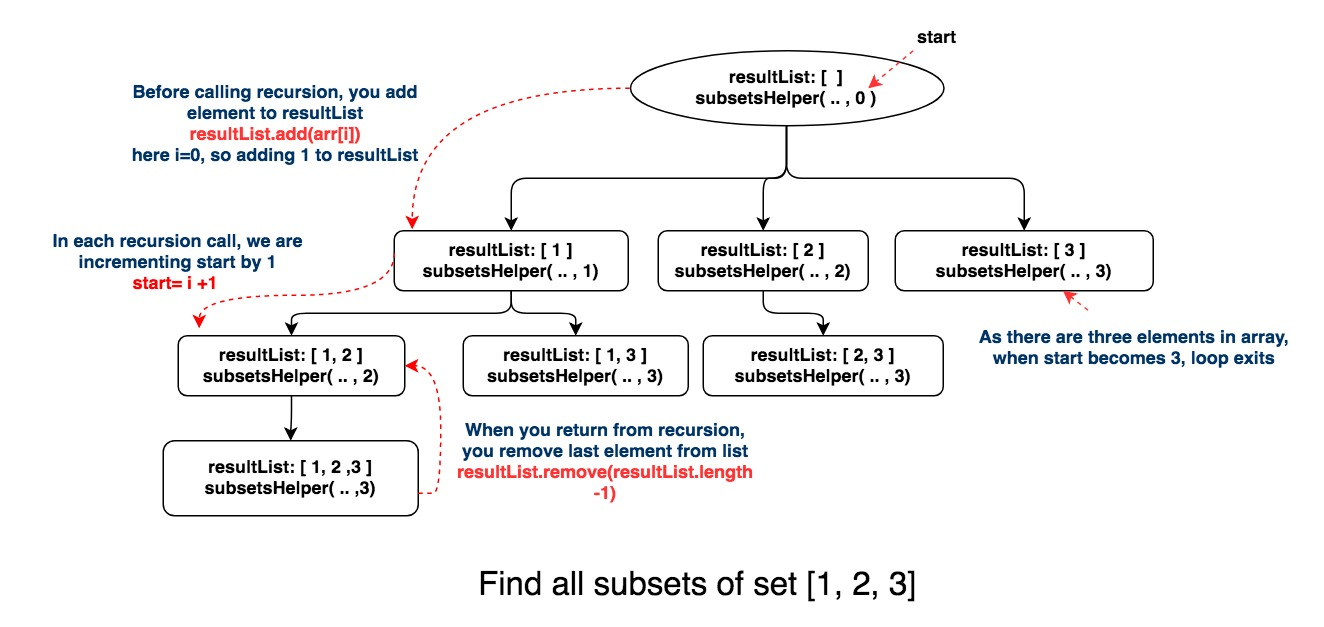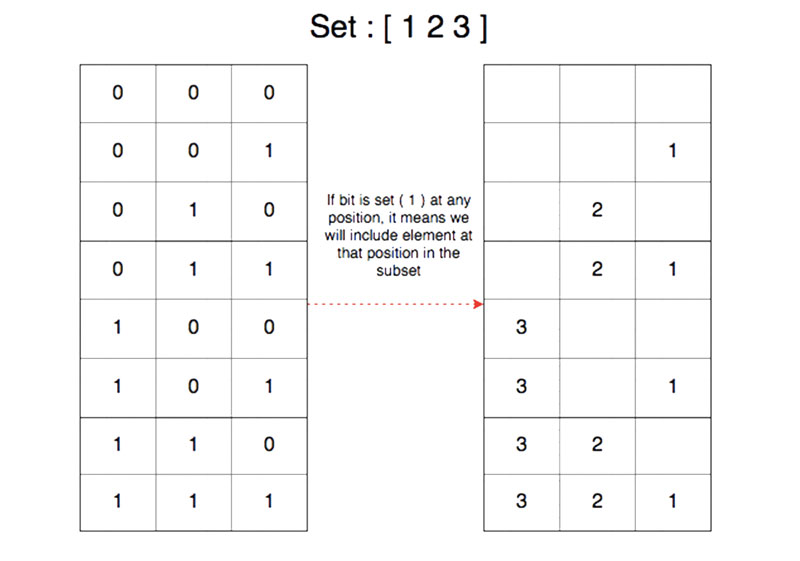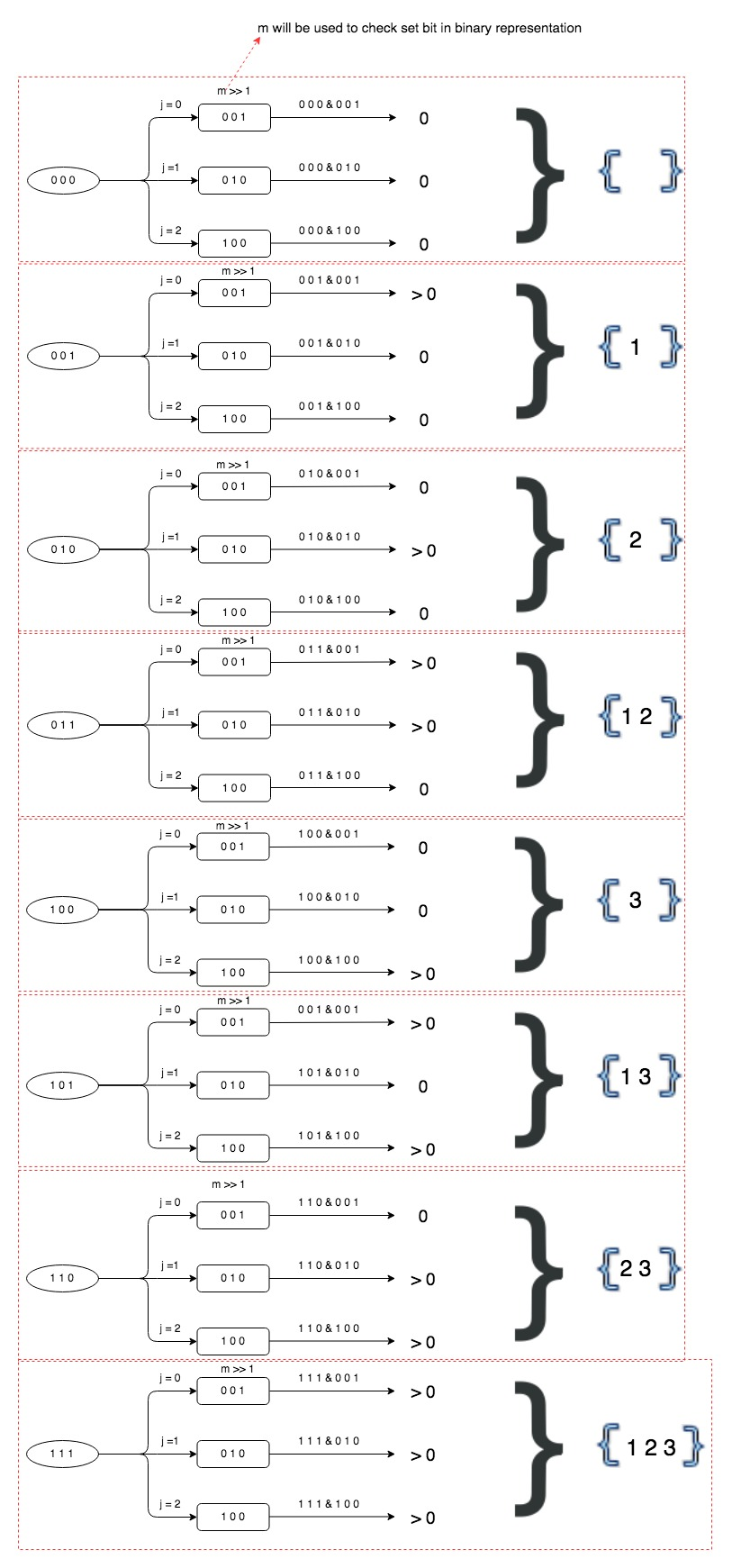# Find all subsets of set (power set) in java

In this post, we will see how to find all subsets of set or power set in java.

## Problem

Given a set of distinct integers, arr, return all possible subsets (the power set).

For example:

Input: nums = [1,2,3]
Output:
[
,
,
,
[1,2,3],
[1,3],
[2,3],
[1,2],
[]
]

We will use two approaches here.

## Using recursion

You can find all subsets of set or power set using recursion. Here is the simple approach.

• As each recursion call will represent subset here, we will add resultList(see recursion code below) to the list of subsets in each call.
• Iterate over elements of a set.
• In each iteration
• Add elements to the list
• explore(recursion) and make start = i+1 to go through remaining elements of the array.
• Remove element from the list.

Here is java code for recursion.

Output

[]

[1, 2]
[1, 2, 1]
[1, 1]

[2, 1]


Let’s understand with the help of a diagram.If you notice, each node(resultList) represent subset here.

## Using bit manipulation

You can find all subsets of set or power set using iteration as well.

There will be 2^N subsets for a given set, where N is the number of elements in set.
For example, there will be 2^4 = 16 subsets for the set {1, 2, 3, 4}.

Each ‘1’ in the binary representation indicate an element in that position.
For example, the binary representation of number 6 is 0101 which in turn represents the subset {1, 3} of the set {1, 2, 3, 4}.How can we find out which bit is set for binary representation, so that we can include the element in the subset?

To check if 0th bit is set, you can do logical & with 1

To check if 1st bit is set, you can do logical & with 2

To check if 2nd bit is set, you can do logical & with 2^2

.

.

To check if nth bit is set, you can do logical & with 2^n.

Let’s say with the help of example:

For a set {1 ,2, 3}

0 1 1  &  0 0 1 = 1    –> 1 will be included in subset

0 1 1  &  0 1 0 = 1    –> 2 will be included in subset

0 1 1  &  1 0  0 =0   –> 3 will not be included in subset.

Here is java code for the bit approach.

Output

{ }
{ 1 }
{ 2 }
{ 1 2 }
{ 3 }
{ 1 3 }
{ 2 3 }
{ 1 2 3 }

Here is complete representation of bit manipulation approach.That’s all about finding all subsets of set(power set).

1.Ujjval February 23, 2020
2.Reejesh October 9, 2020